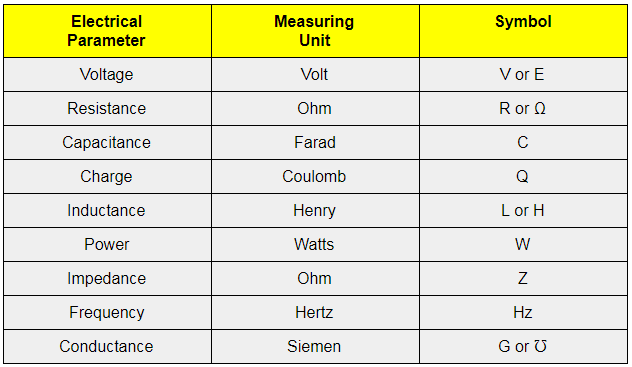# Unit of Electricity

If you have observed electricity bills then the unit of electricity is generally represented in Kilowatt Hour (kWh). Well, the unit represents the actual measure of electricity or the amount of energy that has been used at a property. So if you have been wondering about what is the unit of electricity, the answer is given below.

## SI Unit of Electricity

Talking about the SI unit of electricity, it is represented by the unit of power which is Watt. Interestingly, the name has been kept in honour of James Watt, who is the inventor of the steam engine. When we look at Watt, it mostly equal to one ampere under the pressure of one volt however electricity which is a larger quantity is measured in terms of kilowatt-hours (kWh’s).

### Other Electricity Units

Apart from watt or kilowatt, there are other standard units of measurement used to measure electrical properties like the voltage, resistance, power, capacitance, inductance, and others. Below are some popular electricity units.Related articles: